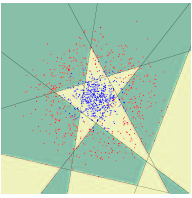## Friday, March 27, 2009

There are a couple of algorithms that float around that I always feel I need to know more about. During a number of data mining conferences the top 10 algorithms in data mining were collated.

One that seems to get a lot of attention in computer vision and robotics is Adaptive Boosting (aka AdaBoost). You can use this algorithm to create a strong classifier from a set of weak classifiers. (One way to think of this is that weak classifiers give you a very approximate answer to a classification problem, strong ones give a much much better approximation)

For example in the figures below, you can see how the algorithm builds up a strong classifier from combining multiple weak classifiers.`Start with a training data set of features, labeled with the correct answer. ie: data[i], correct_answer[i]Run each weak classifier with the data set and store its results.ie:results[x,i] = classifierX(data[i])Initialize each of the weightings with 1 / (number of entries in the data set).At this step we are starting of with each classifier having equal weighting.ie: D[i] = 1/mFor N number of iterations: For each classifier:  For each feature, i:    if the output of the classifier does not equal the correct answer, update the error.    error+=D[i]    (ie: sum of the weights of the misclassified samples)   if the error is less than the minimum error, mark this as the best classifier  End For Now update the importance of the classifier:  alpha[best classifier] = 0.5 * log(1 - minimum error)/minimum error  And update all the classifier weightings: For each feature,i  D[i] = *= exp(-alpha[best] * answer[i] * result(best,i)  z+=D[i]  End for For each feature, update D[i] = D[i]/z to renormalize.`